## Example Questions

### Example Question #6 : Right Triangles

Given a right triangle with a leg length of 6 and a hypotenuse length of 10, find the length of the other leg, x.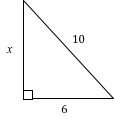4

64

16

8

8

Explanation:

Using Pythagorean Theorem, we can solve for the length of leg x:

x2 + 62 = 102

Now we solve for x:

x2 + 36 = 100

x2 = 100 – 36

x2 = 64

x = 8

Also note that this is proportionally a 3/4/5 right triangle, which is very common. Always look out for a side-to-hypoteneuse ratio of 3/5 or 4/5, or a side-to-side ratio of 3/4, in any right triangle, so that you may solve such triangles rapidly.

### Example Question #7 : Right Triangles

In a right triangle a hypotenuse has a length of 8 and leg has a length of 7. What is the length of the third side to the nearest tenth?

2.4
3.9
1.0
3.6
Explanation:

Using the pythagorean theorem, 82=72+x2. Solving for x yields the square root of 15, which is 3.9

### Example Question #8 : Right Triangles

Given a right triangle with a leg length of 2 and a hypotenuse length of √8, find the length of the other leg, x.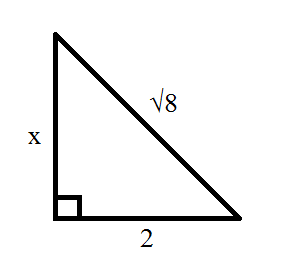10

√8

4

2

6

2

Explanation:

Using Pythagorean Theorem, we can solve for the length of leg x:

x2 + 22 = (√8)2 = 8

Now we solve for x:

x2 + 4 = 8

x2 = 8 – 4

x2 = 4

x = 2

### Example Question #9 : Right Triangles

The legs of a right triangle are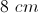and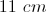. Rounded to the nearest whole number, what is the length of the hypotenuse?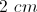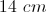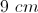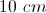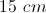Explanation:

Use the Pythagorean Theorem. The sum of both legs squared equals the hypotenuse squared.

### Example Question #141 : Act Math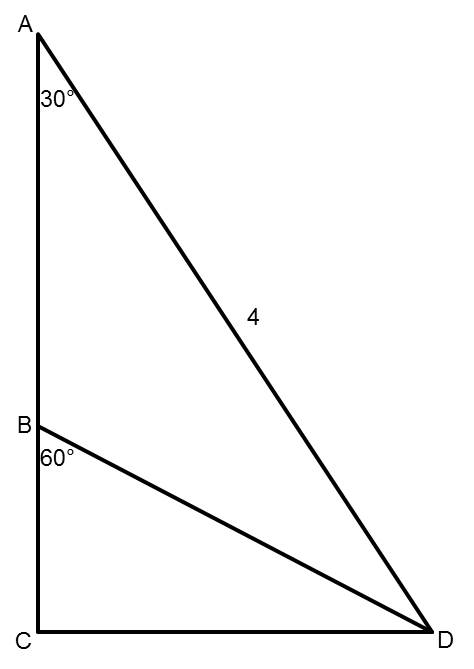Points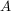,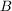, and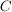are collinear (they lie along the same line).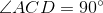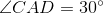,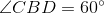,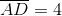Find the length of segment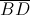.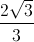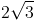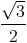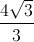Explanation:

The length of segmentisNote that triangles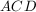and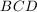are both special, 30-60-90 right triangles. Looking specifically at triangle, because we know that segment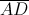has a length of 4, we can determine that the length of segmentis 2 using what we know about special right triangles. Then, looking at trianglenow, we can use the same rules to determine that segmenthas a length of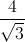which simplifies to.

### Example Question #142 : Act Math

A handicap ramp is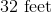long, and a person traveling the length of the ramp goes up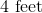vertically. What horizontal distance does the ramp cover?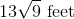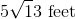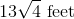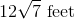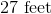Explanation:

In this case, we are already given the length of the hypotenuse of the right triangle, but the Pythagorean formula still helps us. Plug and play, remembering thatmust always be the hypotenuse:State the theorem.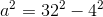Substitute your variables.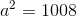Simplify.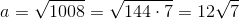Thus, the ramp coversof horizontal distance.